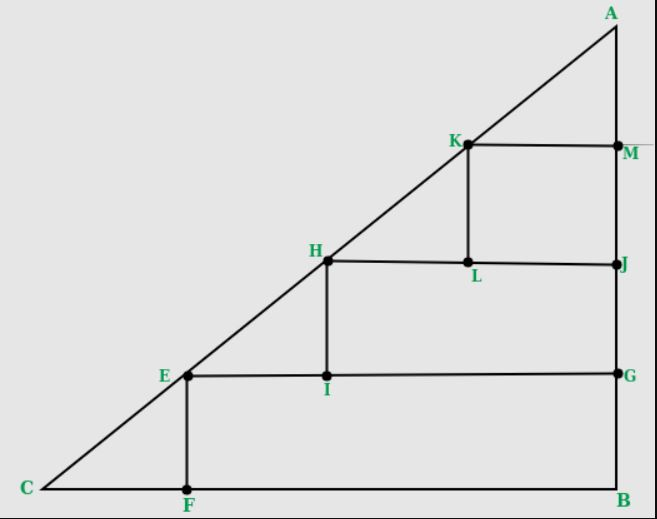GFG App
Open AppBrowser
Continue

# Maximum number of 2×2 squares that can be fit inside a right isosceles triangle

What is the maximum number of squares of size 2×2 units that can be fit in a right-angled isosceles triangle of a given base (in units).
A side of the square must be parallel to the base of the triangle.

Examples:

```Input  : 8
Output : 6
Please refer below diagram for explanation.``````Input : 7
Output : 3```
Recommended Practice

Since the triangle is isosceles, the given base would also be equal to the height. Now in the diagonal part, we would always need an extra length of 2 units in both height and base of the triangle to accommodate a triangle. (The CF and AM segment of the triangle in the image. The part that does not contribute to any square). In the remaining length of base, we can construct length / 2 squares. Since each square is of 2 units, same would be the case of height, there is no need to calculate that again.
So, for each level of given length we can construct “(length-2)/2” squares. This gives us a base of “(length-2)” above it. Continuing this process to get the no of squares for all available “length-2” height, we can calculate the squares.

```while length > 2
answer += (length - 2 )/2
length = length - 2```

For more effective way, we can use the formula of sum of AP n * ( n + 1 ) / 2, where n = length – 2

## C++

 `// C++ program to count number of 2 x 2` `// squares in a right isosceles triangle` `#include` `using` `namespace` `std;`   `int` `numberOfSquares(``int` `base)` `{` `   ``// removing the extra part we would` `   ``// always need` `   ``base = (base - 2);`   `   ``// Since each square has base of` `   ``// length of 2` `   ``base = ``floor``(base / 2);`   `   ``return` `base * (base + 1)/2;` `}`   `// Driver code` `int` `main()` `{` `   ``int` `base = 8;` `   ``cout << numberOfSquares(base);` `   ``return` `0;` `}` `// This code is improved by heroichitesh.`

## Java

 `// Java program to count number of 2 x 2` `// squares in a right isosceles triangle`   `class` `Squares` `{` `  ``public` `static`  `int` `numberOfSquares(``int` `base)` `   ``{` `      ``// removing the extra part ` `      ``// we would always need` `      ``base = (base - ``2``);` ` `  `      ``// Since each square has ` `      ``// base of length of 2` `      ``base = Math.floorDiv(base, ``2``);` ` `  `      ``return` `base * (base + ``1``)/``2``;` `   ``}` ` `  `   ``// Driver code` `   ``public` `static` `void` `main(String args[])` `   ``{` `      `  `      ``int` `base = ``8``;` `      ``System.out.println(numberOfSquares(base));` `   ``}` `}`   `// This code is contributed by Anshika Goyal and improved by heroichitesh.`

## Python3

 `# Python3 program to count number` `# of 2 x 2 squares in a right ` `# isosceles triangle` `def` `numberOfSquares(base):` `    `  `    ``# removing the extra part we would` `    ``# always need` `    ``base ``=` `(base ``-` `2``)` `    `  `    ``# Since each square has base of` `    ``# length of 2` `    ``base ``=` `base ``/``/` `2` `    `  `    ``return` `base ``*` `(base ``+` `1``) ``/` `2` `    `  `# Driver code` `base ``=` `8`   `print``(numberOfSquares(base))`   `# This code is contributed by Anant Agarwal and improved by heroichitesh. `

## C#

 `// C# program to count number of 2 x 2` `// squares in a right isosceles triangle` `using` `System;`   `class` `GFG {` `    `  `    ``public` `static` `int` `numberOfSquares(``int` `_base)` `    ``{` `        `  `        ``// removing the extra part ` `        ``// we would always need` `        ``_base = (_base - 2);` `    `  `        ``// Since each square has ` `        ``// base of length of 2` `        ``_base = _base / 2;` `    `  `        ``return` `_base * (_base + 1)/2;` `    ``}` `    `  `    ``// Driver code` `    ``public` `static` `void` `Main()` `    ``{` `        `  `        ``int` `_base = 8;` `        ``Console.WriteLine(numberOfSquares(_base));` `    ``}` `}`   `// This code is contributed by anuj_67.`

## PHP

 ``

## Javascript

 ``

Output:

`6`

Time complexity : O(1)

Auxiliary Space : O(1)

This article is contributed by Harshit Agrawal. If you like GeeksforGeeks and would like to contribute, you can also write an article using write.geeksforgeeks.org or mail your article to review-team@geeksforgeeks.org. See your article appearing on the GeeksforGeeks main page and help other Geeks.